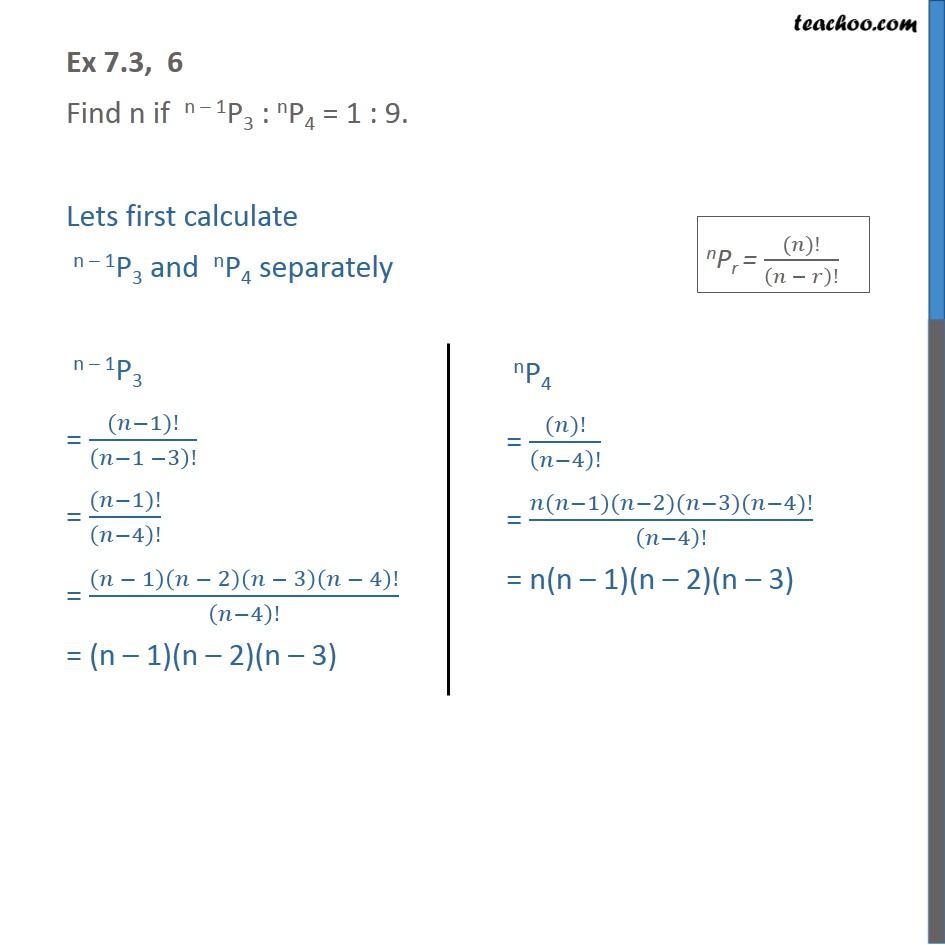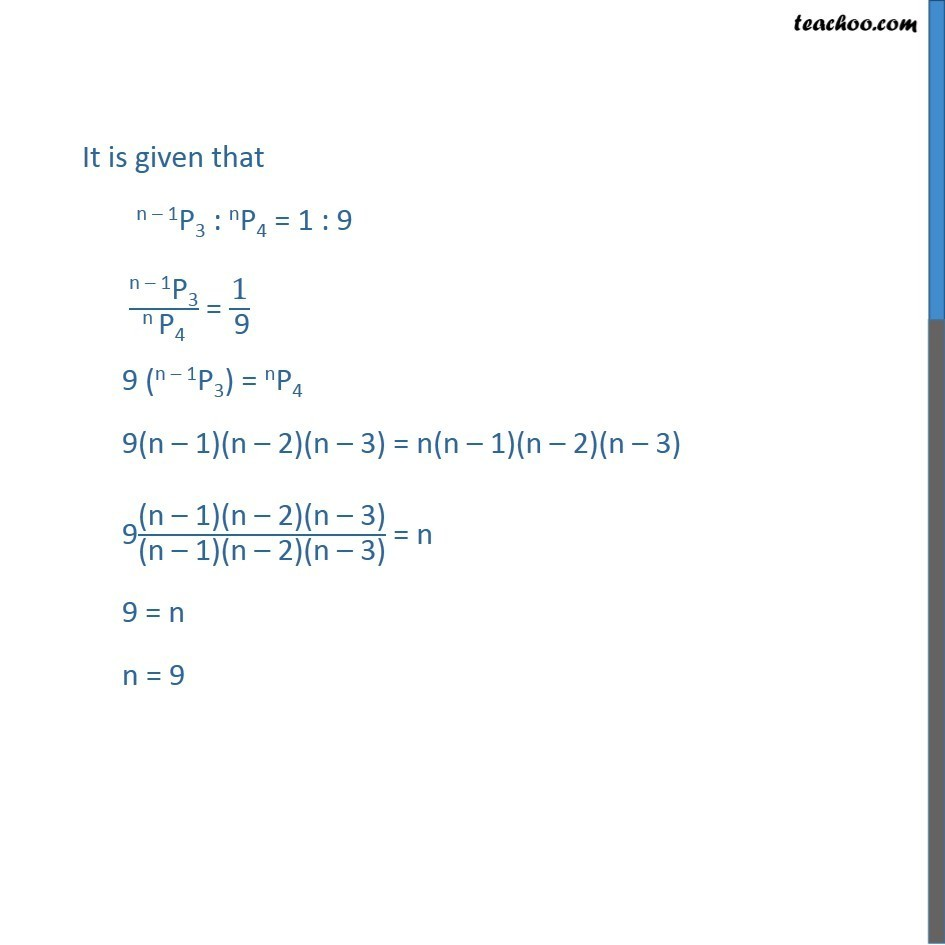1. Chapter 7 Class 11 Permutations and Combinations (Term 2)
2. Serial order wise
3. Ex 7.3

Transcript

Ex 7.3, 6 Find n if n – 1P3 : nP4 = 1 : 9. Lets first calculate n – 1P3 and nP4 separately n – 1P3 = ((𝑛−1)!)/(𝑛−1 −3)! = ((𝑛−1)!)/(𝑛−4)! = (𝑛 − 1)(𝑛 − 2)(𝑛 − 3)(𝑛 − 4)!/(𝑛−4)! = (n – 1)(n – 2)(n – 3) It is given that n – 1P3 : nP4 = 1 : 9 "n – 1P3" /"n P4" = "1" /" 9" 9 (n – 1P3) = nP4 9(n – 1)(n – 2)(n – 3) = n(n – 1)(n – 2)(n – 3) 9"(n – 1)(n – 2)(n – 3)" /"(n – 1)(n – 2)(n – 3)" = n 9 = n n = 9